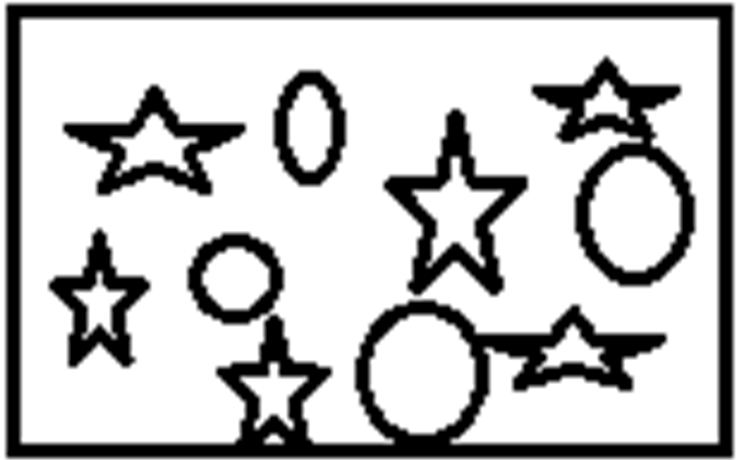# Concept Of Ratio

The concept of ratio and proportion explains how to solve ratios, types of ratios, ratio formula, etc. A ratio is a mathematical expression of comparing two similar or different quantities by division. This expression can be expressed from ratio to percentage form by conversion method. It is denoted by ‘:’ symbol. For example, 2:3, 5:6, 3:7, etc. are ratios. It is also represented by ‘/’ symbol. Hence, the ratio is basically a type of division method.

It helps one to compare two things of the same dimensions or of different dimensions.  For example, in a box, there are 9 red balls and 12 black balls. When we have to find the ratio of red balls to black balls, it means how many numbers of red balls are there as compared to black balls. Here, a number of red balls to black balls are written in form 9:12 (i.e. nine is to twelve) which is same as 3:4 (simplified form). It tells how much amount of the first quantity is there as compared to that of the second quantity.

## How to Solve Ratios?

Several quantities such as height, weight, length, objects, population, etc can be compared in terms of ratio. Generally, it is expressed using the word “to” i.e. such as red balls to black balls. There are other two notations of expressing it, one by using “:” (9:12) which is called odds notation and another one is fractional notation using “/” (9/12). Another important thing to be followed is the order.  When we say the ratio of red balls to black balls, the number representing red ball must come first i.e. 9:12 is not same as 12:9.

It compares not only the quantities of similar dimensions but also that of different dimensions. For example, women are to men in a population, boys are to girls in a class etc. are a comparison of the same dimensions while speed is to time is a comparison of two different dimensions.

Let us solve an example here.

Solve: From the figure below, compare the number of stars to circles in the given rectangle.Solution: The number of stars in the rectangle=6

The number of circles in the rectangle=4

Star is to circle= 6:4 = 3:2

## Types of Ratios in Math

There are various types of ratios in Maths. They are:

• Compounded Ratio: The compounded ratio of the two ratios a : b and c : d is the ratio ac : bd, and that of a : b, c : d and e : f is the ratio ace : bdf.
• Duplicate Ratio: The duplicate ratio of the ratio a : b is the ratio a2 : b2
• Reciprocal Ratio: The reciprocal ratio of a:b is (1/a):(1/b), where a≠0 and b≠0
• Ratio of equalities: If the antecedent and consequent are equal then the ratio is called ratio of equality, like 6:6.
• Ratio of Inequalities: If the antecedent and consequent are not equal then the ratio is called the ratio of inequality, like 4:7.

### Ratio and Proportion Examples

Numbers are inevitable and life without numbers is unthinkable. So is the ratio; it has various real-time applications which you find in daily life. The most common example is cooking; the ratio of ingredients has to be maintained for a good dish. Other fields where we find its applications are in business, sports, construction of buildings and so on.

To solve more problems on the topic, download BYJU’S – The Learning App and watch interactive videos. Also, take free tests to practice for exams.